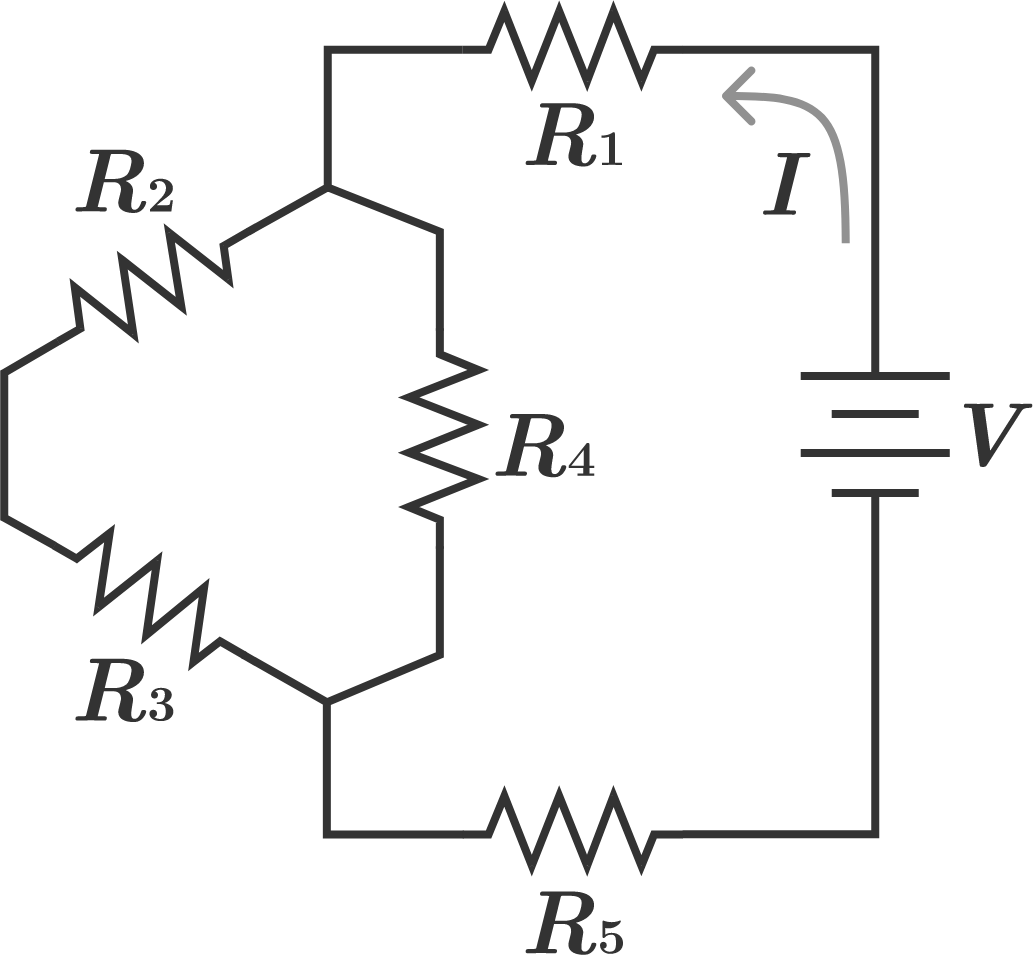# Circuit ResistanceA $30 \text{V}$ battery $V$ is connected to a five resistor $R$ circuit. Each of five resistor has a resistance of $9 \Omega$. Calculate the current $I$ in amperes.

###### David's Electricity Set
×

Problem Loading...

Note Loading...

Set Loading...